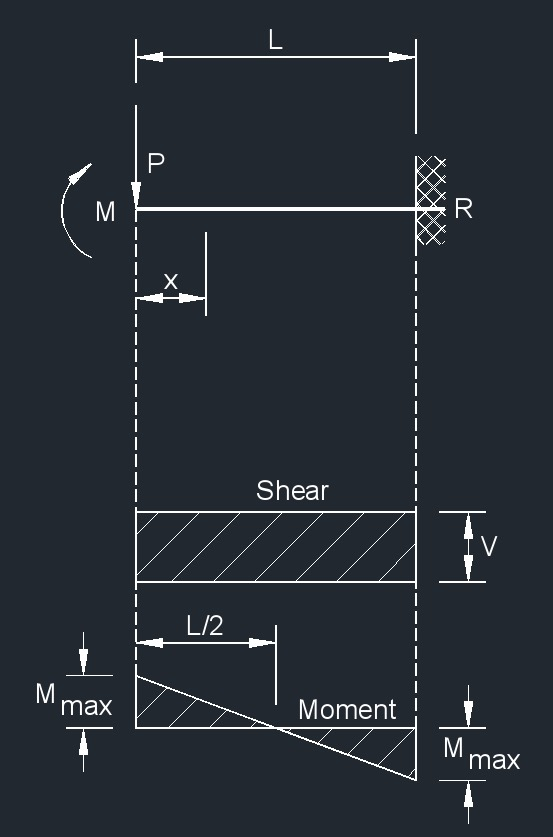# Cantilever Beam - Load at Free End Deflection Vertically with No Rotation

Written by Jerry Ratzlaff on . Posted in Structural

###Cantilever Beam - Load at Free End Deflection Vertically with No Rotation Formula

$$\large{ R = V = P }$$

$$\large{ M_{max} \; }$$   (at both end)   $$\large{ = \frac{P L}{2} }$$

$$\large{ M_x = P \left( \frac{ L }{2} - x \right) }$$

$$\large{ \Delta_{max} \; }$$   (at free end)   $$\large{ = \frac{P L^3}{12 \lambda I} }$$

$$\large{ \Delta_x = \frac{P \left( L -x \right)^2 }{12 \lambda I} \left( L + 2x \right) }$$

Where:

$$\large{ I }$$ = moment of inertia

$$\large{ L }$$ = span length of the bending member

$$\large{ M }$$ = maximum bending moment

$$\large{ P }$$ = total concentrated load

$$\large{ R }$$ = reaction load at bearing point

$$\large{ V }$$ = shear force

$$\large{ w }$$ = load per unit length

$$\large{ W }$$ = total load from a uniform distribution

$$\large{ x }$$ = horizontal distance from reaction to point on beam

$$\large{ \lambda }$$   (Greek symbol lambda) = modulus of elasticity

$$\large{ \Delta }$$ = deflection or deformation